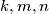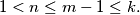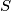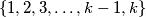### IMO Shortlist 1996 problem C3

Kvaliteta:
Avg: 0,0
Težina:
Avg: 7,0
Let$k,m,n$ be integers such that$1 < n \leq m - 1 \leq k.$ Determine the maximum size of a subset$S$ of the set$\{1,2,3, \ldots, k-1,k\}$ such that no$n$ distinct elements of$S$ add up to$m.$
Izvor: Međunarodna matematička olimpijada, shortlist 1996